# Discuss the shape of the following molecules using the VSEPR model:

Question:

Discuss the shape of the following molecules using the VSEPR model:

$\mathrm{BeCl}_{2}, \mathrm{BCl}_{3}, \mathrm{SiCl}_{4}, \mathrm{ASF}_{5}, \mathrm{H}_{2} \mathrm{~S}, \mathrm{PH}_{3}$

Solution:

$\mathrm{BeCl}_{2}:$

$\mathrm{Cl}: \mathrm{Be}: \mathrm{Cl}$

The central atom has no lone pair and there are two bond pairs. i.e., $\mathrm{BeCl}_{2}$ is of the type $\mathrm{AB}_{2} . \mathrm{Hence}$, it has a linear shape.

$\mathrm{BCl}_{3}$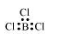The central atom has no lone pair and there are three bond pairs. Hence, it is of the type $\mathrm{AB}_{3}$. Hence, it is trigonal planar.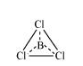$\mathrm{SiCl}_{4:}$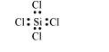The central atom has no lone pair and there are four bond pairs. Hence, the shape of $\mathrm{SiCl}_{4}$ is tetrahedral being the $\mathrm{AB}_{4}$ type molecule.

$\underline{\mathrm{ASF}}_{5}:$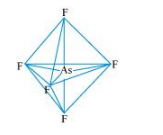The central atom has no lone pair and there are five bond pairs. Hence, $\mathrm{AsF}_{5}$ is of the type $\mathrm{AB}_{5}$. Therefore, the shape is_trigonal bipyramidal.

$\mathrm{H}_{2} \mathrm{~S}$The central atom has one lone pair and there are two bond pairs. Hence, $\mathrm{H}_{2} \mathrm{~S}$ is of the type $\mathrm{AB}_{2} \mathrm{E}$. The shape is Bent.

$\mathrm{PH}_{3}:$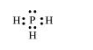The central atom has one lone pair and there are three bond pairs. Hence, $\mathrm{PH}_{3}$ is of the $\mathrm{AB}_{3} \mathrm{E}$ type. Therefore, the shape is trigonal pyramidal.